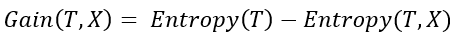Classification

Decision Tree

Machine Learning

Regression

January 8, 2021

# What do you understand by Information Gain? How does it help in tree building?

information gain is equal to reduction in entropy. It measures the amount of reduction in randomness/entropy after decision tree has made the split. It is the difference in entropies before and after the split.Where, T is the parent node before split and X is the split node from T.

Information Gain is the cost function that decision trees employ as basis of splitting the data, if the the split leads to increase in Information Gain then it’s carried out else not.

by : Monis Khan

Quick Summary:

information gain is equal to reduction in entropy. It measures the amount of reduction in randomness/entropy after decision tree has made the split. It is the difference in entropies before and after the split. Where, T is the parent node before split and X is the split node from T. Information Gain is the cost […]